First of all, let’s understand what is a timer task? Timers have very many scenarios, which you should encounter frequently in your normal work, such as generating monthly statistical reports, financial reconciliation, membership points settlement, email push, etc., are all scenarios of timer usage. Timers generally have three forms of performance: execution at a fixed period, execution after a certain delay, and execution at a specified time.

The essence of a timer is to design a data structure that can store and schedule a collection of tasks, and the closer the deadline, the higher the priority of the task. So how does a timer know if a task is due or not? A timer needs to be polled to check if a task is due every other time slice.

So the internal structure of a timer typically requires a task queue and an asynchronous polling thread, and can provide three basic operations.

• Run executes a task that is due.

The JDK natively provides three common timer implementations, namely Timer, DelayedQueue and ScheduledThreadPoolExecutor, which are described in turn.

Timer

Timer is a relatively early implementation of the JDK that allows for fixed-period tasks, as well as deferred tasks; Timer starts an asynchronous thread to execute a task when it is due, and the task can be scheduled only once, or repeated several times periodically. Let’s look at how the Timer is used, the example code is as follows.

 ``````1 2 3 4 5 6 7 `````` ``````Timer timer = new Timer(); timer.scheduleAtFixedRate(new TimerTask() { @Override public void run() { // do something } }, 10000, 1000); // 10s 后调度一个周期为 1s 的定时任务 ``````

We can see that the task is implemented by the TimerTask class, which is an abstract class that implements the Runnable interface, and the Timer is responsible for scheduling and executing the TimerTask.

 ``````1 2 3 4 5 6 7 8 `````` ``````public class Timer { private final TaskQueue queue = new TaskQueue(); private final TimerThread thread = new TimerThread(queue); public Timer(String name) { thread.setName(name); thread.start(); } } ``````

TaskQueue is a rootlet heap implemented as an array structure, with the deadline nearest task at the top of the heap and queue always being the highest priority task to be executed. So using the rootlet heap data structure, the time complexity of the Run operation is O(1), and the time complexity of both the Add Schedule and Cancel operations is O(logn).

DelayedQueue

DelayedQueue is a blocking queue in the JDK that can delay the fetching of objects and internally stores objects using a priority queue, PriorityQueue. DelayedQueue is used in the following way

 `````` 1 2 3 4 5 6 7 8 9 10 11 12 13 14 15 16 17 18 19 20 21 22 23 24 25 26 27 28 29 30 31 32 33 `````` ``````public class DelayQueueTest { public static void main(String[] args) throws Exception { BlockingQueue delayQueue = new DelayQueue<>(); long now = System.currentTimeMillis(); delayQueue.put(new SampleTask(now + 1000)); delayQueue.put(new SampleTask(now + 2000)); delayQueue.put(new SampleTask(now + 3000)); for (int i = 0; i < 3; i++) { System.out.println(new Date(delayQueue.take().getTime())); } } static class SampleTask implements Delayed { long time; public SampleTask(long time) { this.time = time; } public long getTime() { return time; } @Override public int compareTo(Delayed o) { return Long.compare(this.getDelay(TimeUnit.MILLISECONDS), o.getDelay(TimeUnit.MILLISECONDS)); } @Override public long getDelay(TimeUnit unit) { return unit.convert(time - System.currentTimeMillis(), TimeUnit.MILLISECONDS); } } } ``````

The DelayQueue provides the put() and take() blocking methods to add objects to and take objects out of the queue. Once an object has been added to the DelayQueue, it is prioritised according to the compareTo() method. getDelay() is used to calculate the time left for the message to be delayed and the object can only be taken out of the DelayQueue if getDelay <= 0.

The most common scenario where DelayQueue is used in everyday development is to implement a retry mechanism. For example, if an interface call fails or a request times out, the current request object can be put into the DelayQueue, taken out via an asynchronous thread take() and retried. To limit the frequency of retries, you can set a maximum number of retries and set the object’s deadline using an exponential fallback algorithm, e.g. 2s, 4s, 8s, 16s …… and so on.

DelayQueue uses a priority queue to prioritise tasks and the time complexity of adding a Schedule and cancelling a Cancel operation is also O(logn).

The Timer described above is actually not recommended for users at the moment, it has a number of design flaws.

• The Timer is a single-threaded model. If a TimerTask takes a long time to execute, it will affect the scheduling of other tasks.
• Timer’s task scheduling is based on absolute system time, which may cause problems if the system time is incorrect.
• Timer does not catch an exception if a TimerTask executes, which can cause the thread to terminate and other tasks to never execute.

To address the design flaws of the Timer, the JDK provides the more feature-rich ScheduledThreadPoolExecutor, which offers both periodic and deferred execution of tasks. Here is an example of how the ScheduledThreadPoolExecutor can be used.

 ``````1 2 3 4 5 6 `````` ``````public class ScheduledExecutorServiceTest { public static void main(String[] args) { ScheduledExecutorService executor = Executors.newScheduledThreadPool(5); executor.scheduleAtFixedRate(() -> System.out.println("Hello World"), 1000, 2000, TimeUnit.MILLISECONDS); // 1s 延迟后开始执行任务，每 2s 重复执行一次 } } ``````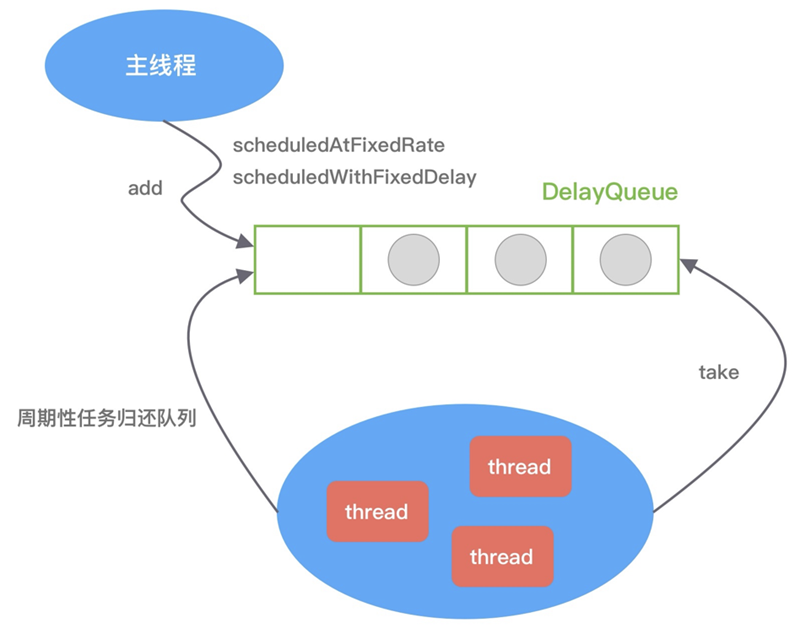Above we have briefly described the three ways in which the JDK implements timers. We can say that their implementation ideas are very similar, all of them are inseparable from the three roles of task, task management and task scheduling. The time complexity of adding and removing tasks is O(nlog(n)), and all three timers will encounter serious performance bottlenecks in the face of massive task insertion and deletion scenarios. Therefore, for scenarios with high performance requirements, we generally use the time wheel algorithm.

## Time Wheel Principle

If there are a large number of scheduled tasks in a system, and each of the large number of scheduled tasks uses its own scheduler to manage the life cycle of the task, it is wasteful of cpu resources and inefficient. The time wheel is a scheduling model that makes efficient use of thread resources for batch scheduling. It binds a large number of scheduled tasks to a single scheduler and uses this scheduler to manage, trigger and runnable all tasks. This allows for efficient management of various delayed tasks, periodic tasks, notification tasks, etc.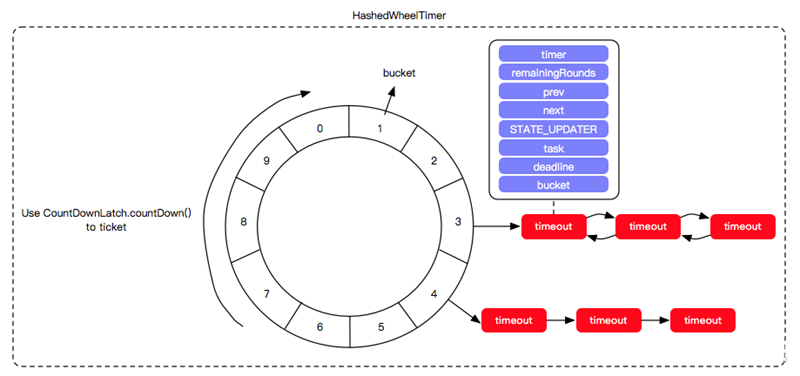The core of the time wheel algorithm is that the polling thread is no longer responsible for traversing all the tasks, but only the time scale. The time wheel algorithm is like a hand constantly rotating and traversing a clock, and if one finds a task (task queue) on it at a certain moment, then it will execute all the tasks on the task queue.

The time wheel algorithm no longer uses the task queue as a data structure, which is shown in the following diagram (we will use the hour as a unit).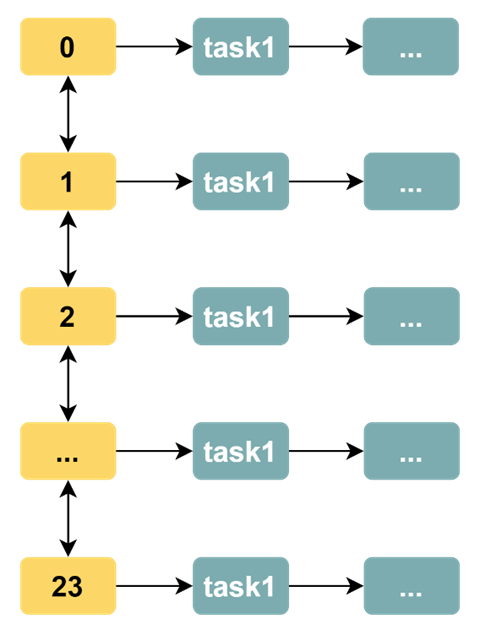Obviously, the time-wheel algorithm solves the problem of inefficient traversal. In the time wheel algorithm, the polling thread always executes all the tasks in the task queue on the corresponding scale after it has traversed a time scale (usually by throwing the tasks to the asynchronous thread pool for processing), instead of traversing to check that the timestamps of all tasks are met.

Now, even if there are 10k tasks, the polling thread does not have to iterate over 10 k tasks per round, but only over 24 time scales.

A time wheel algorithm in hours is thus simply implemented. However, the hour as a unit of time is too granular and we sometimes want to base the time scale on minutes. The most straightforward way to do this is to increase the time scale so that each day has 24 * 60 = 1440. The data structure of the time wheel would then be as follows.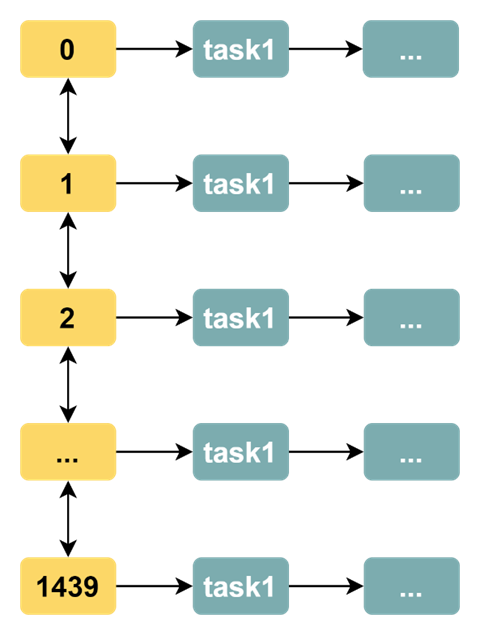By adding a time scale, we can perform timed tasks based on finer units of time (minutes). However, this implementation has the following drawbacks.

• Inefficient polling thread traversal problem: when the number of timescales increases and the number of tasks is small, the efficiency of the polling thread traversal decreases, e.g. if there are tasks on only 50 timescales but 1440 timescales need to be traversed. this defeats the original purpose of our time wheel algorithm: to solve the problem of inefficient traversal of the traversal polling threads.
• The problem of wasted memory space: with dense timescales and a small number of tasks, the memory space occupied by most timescales is meaningless.

If the time precision were to be set to seconds, then the entire time wheel would require 86,400 units of time ticks, at which point the traversal threads of the time wheel algorithm would encounter even greater operational inefficiencies.

## Hierarchical time wheel algorithm

The hierarchical time wheel algorithm has its counterpart model in life, that of the water meter: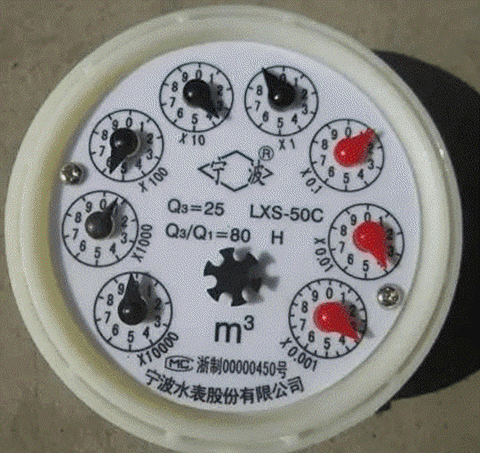At this point, we have three time wheels at the second, minute and hour levels, each with 60, 60 and 24 scales respectively.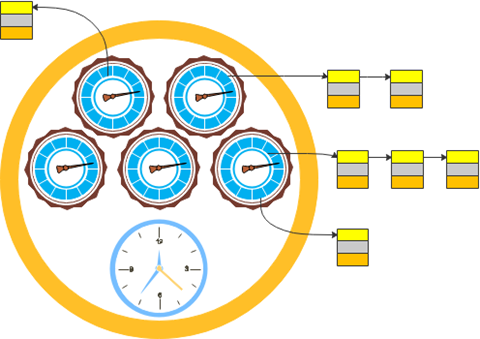The layered time wheel is shown in the following diagram.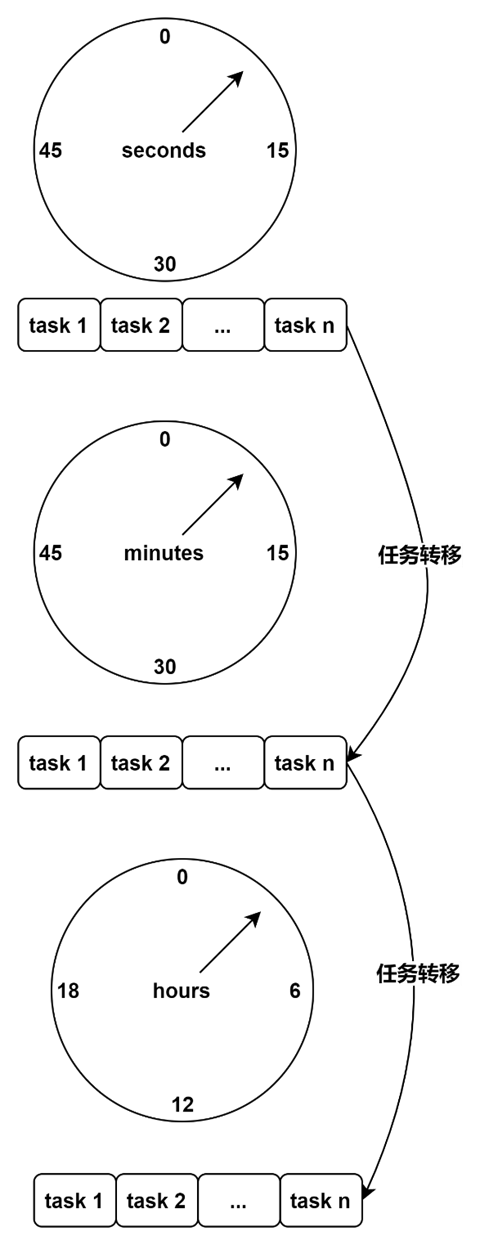Suppose our task needs to be executed once a day at 7:30:20 seconds. The task is first added to tick 20 of the seconds clock wheel, and when its polling thread visits tick 20, it is transferred to tick 30 of the minutes clock wheel. When the minute level clock wheel thread visits tick 30, it transfers this task to tick 7 of the hour level clock wheel. When the 7th tick is accessed by the hourly clock wheel thread, the task is finally handed over to the asynchronous thread for execution, and then the task is registered again in the second-level time wheel.

The transfer of tasks from one time wheel to another in the hierarchical time wheel is similar to the way a turn of a small unit of a water meter causes a higher unit of the meter to advance one unit.

Since time wheels exist in components such as Netty, Akka, Quartz, ZooKeeper, Kafka, etc., a detailed explanation of the implementation and usage will not be given here.